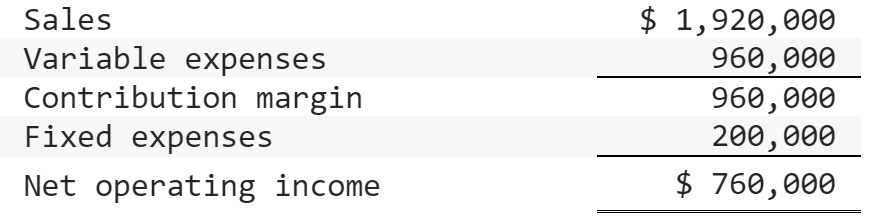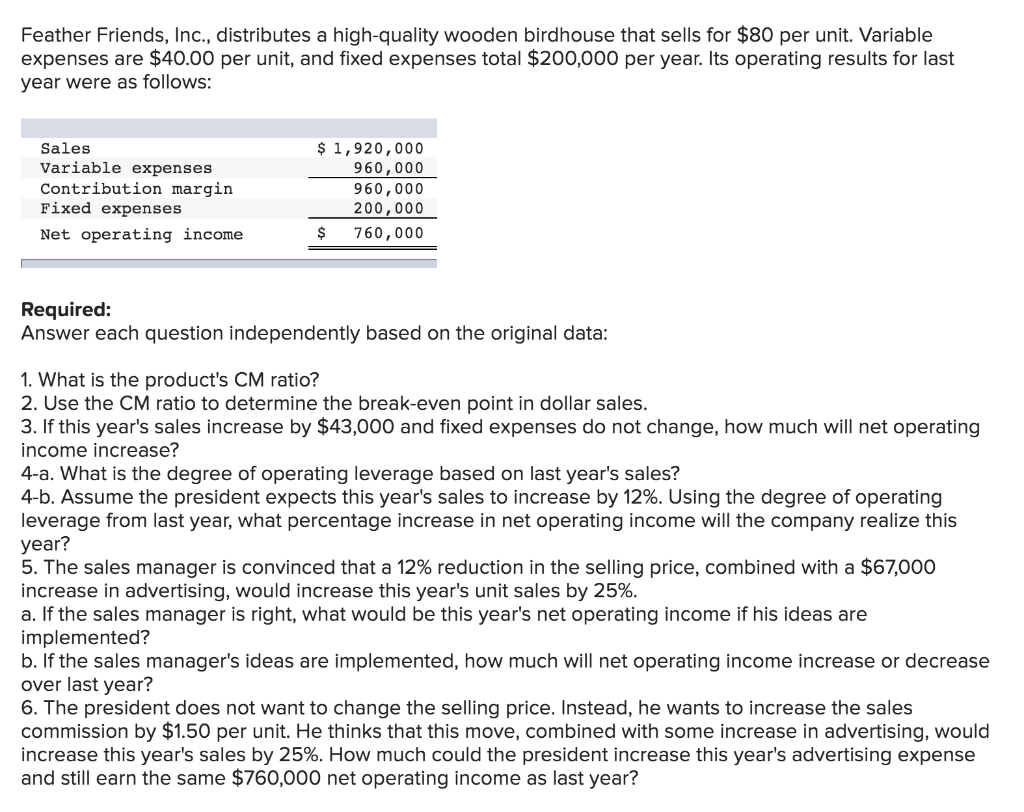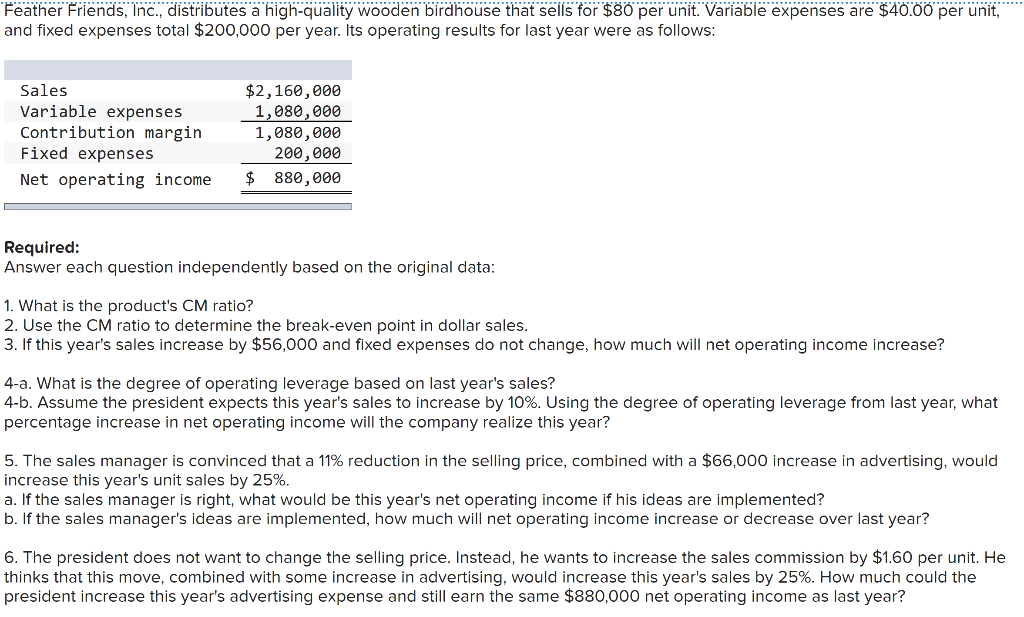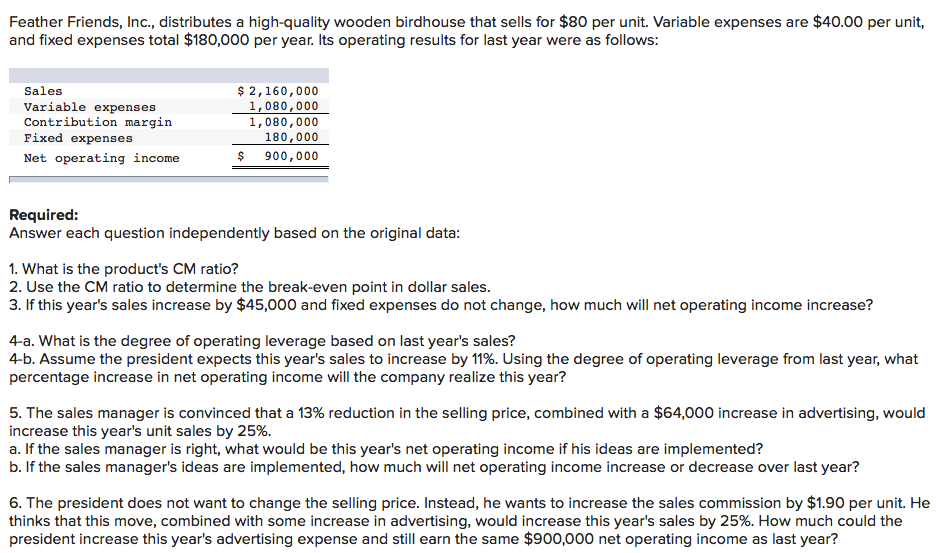Question

# Feather Friends, Incorporated, distributes a high-quality wooden birdhouse that sells for \$80 per unit. Variable expenses are \$40.00 per unit, and fixed expenses total \$200,000 per year. Its operating results for last year were as follows:

Feather Friends, Incorporated, distributes a high-quality wooden birdhouse that sells for \$80 per unit. Variable expenses are \$40.00 per unit, and fixed expenses total \$200,000 per year. Its operating results for last year were as follows:Required:

Answer each question independently based on the original data:

1. What is the product's CM ratio?

2. Use the CM ratio to determine the break-even point in dollar sales.

3. Assume this year’s unit sales and total sales increase by 49,000 units and \$3,920,000, respectively. If the fixed expenses do not change, how much will net operating income increase?

4-a. What is the degree of operating leverage based on last year's sales?

4-b. Assume the president expects this year's unit sales to increase by 10%. Using the degree of operating leverage from last year, what percentage increase in net operating income will the company realize this year?

5. The sales manager is convinced that a 11% reduction in the selling price, combined with a \$78,000 increase in advertising, would increase this year's unit sales by 25%.

a. If the sales manager is right, what would be this year's net operating income if his ideas are implemented?

b. If the sales manager's ideas are implemented, how much will net operating income increase or decrease over last year?

6. The president does not want to change the selling price. Instead, he wants to increase the sales commission by \$2.30 per unit. He thinks that this move, combined with some increase in advertising, would increase this year's unit sales by 25%. How much could the president increase this year's advertising expense and still earn the same \$760,000 net operating income as last year?

1)

contribution margin ratio = contribution margin per unit ÷ sales price per unit

= [960000÷1920000/80] ÷ 80

= 40 ÷ 80

= 0.5 or 50%

2)

break even points (dollar sales ) =fixed cost ÷ contribution margin ratio

= \$ 200000 ÷ 50%

= \$ 400000

3)

if the sales increase by 56000 units or \$ 4480000

increase in operating income = increase in sales * contribution margin ratio

= \$ 4480000 * 50%

= \$ 2240000

4)a)

Degree of operating leverage = contribution margin ÷ operating income

= 960000 ÷ 760000

= 1.2631578947368421052631578947368 or 1.26 approx

4)b)

% increase in operating income = % increase in sales * operating leverage

= 14 % * 1.2631578947368421052631578947368

= 17.684210526315789473684210526315 % or 17.68 % approx

5)

a) Computation of net operating income:

Sales units = Sales ÷ Unit selling price = = \$1,920,000 ÷ \$80 = 24,000 units

Revised sales units = 24,000 + (24,000 * 25%) = 30,000 units

Revised unit selling price = \$80 – (\$80 * 15%) = \$68 per unit

 Sales (30,000 units * \$68) \$2,040,000 Less: Variable exps (30,000 * \$40) 1,200,000 Contribution margin (CM) 840,000 Less: Fixed costs (\$200,000 + \$60,000) 260,000 Net operating income \$580,000

b) Last year operating income was \$760,000 and based on the suggestion of sales manager current year net operating income would be \$580,000. So if the sales manager's ideas are implemented, net operating income will be reduced by \$180,000.

6)

 Sales (30,000 units * \$80) \$2,400,000 Less: Variable exps [30,000 units * (\$40 + \$1.80)] 1,254,000 CM \$1,146,000

Net operating income = CM – Fixed costs

\$760,000 = \$1,146,000 – (\$200,000 + Incremental advertising exps)

Therefore, advertising exps. can be increased by \$186,000 so that the net operating income remains at \$760,000 as last year.

SOLUTION :

1.

Sale price per unit, P                  = \$80

Variable cost per unit , VC         = \$40

=> CM per unit (UCM) = 80 - 40  = \$40

CM ratio

= UCM/P = 40/80

2.

Fixed costs, FC = \$200000

BEP in dollars sales

= FC / CM ratio

= 200000/0.5

3.

Present sales = 1920000 (\$)

It is quite above the BEP sales.

So any further increase in sales will result in increase of TCM.

The entire increase in. TCM will go to increase in operating income.

So,

Increase in operating income

= Increase in TCM

(since FC is already covered by present sales volume)

= Increase in sales * CM ratio

= 3920000 * 0.5

= 1960000 (\$) (NSWER).

4 a.

DOL (based on last year’s sales)

= TCM / Operating income

= 960000 / 760000

4 b.

% increase in OI

= % increase in sales * DOL

= 10% * 1.263

5.

a.

New CM

= New sales - last year’s VC

= 1920000*1.25*(1 - 0.11)* - 960000*1.25

= 936000 (\$)

New FC = 200000 + 78000 = 278000 )\$)

Net OI

= New CM  - New FC

= 936000 - 278000

b.

% change in OI

= (New OI - Previous OI) / Previous OI  *100

= (658000 - 760000)/760000 *100

= - 13.42% (decrease of OI)  (ANSWER).

6.

New CM

= New sales - New VC

= 1920000*1.25 - (1920000*1.25/80 * (40+2.30))

= 1131000 (\$)

New FC = 200000 + Advt. expenses increase, A)

New OI = 1131000 - (200000 + A)

=> 760000 = 931000 - A

=> A = increase of Advt. expense

= 9310000 - 760000

 Req 1 Product CM Ratio 50.00% Note 1: CM Ratio = Contribution margin / sales Contribution margin 960,000 Divided by sales 1,920,000 CM Ratio 50.00% Req 2 Break Even points in sales dollar 400,000 Note 2: Break Even points in sales dollar = Fixed expense/CM Ratio Fixed expenses 200,000 Divided by CM Ratio 50.00% Break Even points in sales dollar 400,000 Req 3 Net Operating Income Increase by 1,960,000 Note 3: Effect on income = change in sales * CM Ratio Increase in sales 3,920,000 Multiply by CM Ratio 50% Net Operating Income Increase by 1,960,000 Req 4-a Degree of Operating Leverage 1.26 Note 4: Operating Leverage = Contribution margin/ Net Income Contribution Margin 960,000 Divided by Net Income 760,000 Degree of Operating Leverage 1.26 Req 4-b Net Operating Income(NOI) increase by 12.60% Note 5: Change in NOI = %age change in sales * operating leverage %age change in sales 10% Multiply by operating leverage 1.26 Net Operating Income(NOI) increase by 12.60% Req 5-a Contribution Income   statement Last Year Proposed 24,000 Units 30,000 Units Total Per Unit Total Per Unit Sales 1,920,000 80.00 2,136,000 71.20 Less: Variable expense 960,000 40.00 1,200,000 40.00 Contribution margin 960,000 40.00 936,000 31.20 Fixed expenses 200,000 278,000 Net Income 760,000 658,000 Req 5-b Net Operating Income Decrease by (102,000) Req 6 The amount by which advertising can be increased 171,000 Note6: Contribution margin per unit (Last year) 40.00 Less: increase in sales comm (2.30) Prposed contribution margin 37.70 Multiplied by proposed sales after increase 30,000 Proposed total contribution margin 1,131,000 Less: Fixed expenses (200,000) Less: Target Income (760,000) The amount by which advertising can be increased 171,000

source: managerial accounting

#### Earn Coins

Coins can be redeemed for fabulous gifts.

Similar Homework Help Questions
• ### Feather Friends, Inc., distributes a high-quality wooden birdhouse that sells for \$80 per unit. Variable expenses...

Feather Friends, Inc., distributes a high-quality wooden birdhouse that sells for \$80 per unit. Variable expenses are \$40.00 per unit, and fixed expenses total \$180,000 per year. Its operating results for last year were as follows: Sales \$ 2,000,000 Variable expenses 1,000,000 Contribution margin 1,000,000 Fixed expenses 180,000 Net operating income \$ 820,000 Required: Answer each question independently based on the original data: 1. What is the product's CM ratio? 2. Use the CM ratio to determine the break-even point...

• ### Feather Friends, Inc., distributes a high-quality wooden birdhouse that sells for \$80 per unit. Variable expenses...

Feather Friends, Inc., distributes a high-quality wooden birdhouse that sells for \$80 per unit. Variable expenses are \$40.00 per unit, and fixed expenses total \$180,000 per year. Its operating results for last year were as follows: Sales \$ 2,160,000 Variable expenses 1,080,000 Contribution margin 1,080,000 Fixed expenses 180,000 Net operating income \$ 900,000 Required: Answer each question independently based on the original data: 1. What is the product's CM ratio? 2. Use the CM ratio to determine the break-even point...

• ### Feather Friends, Inc., distributes a high-quality wooden birdhouse that sells for \$80 per unit. Variable expenses...

Feather Friends, Inc., distributes a high-quality wooden birdhouse that sells for \$80 per unit. Variable expenses are \$40.00 per unit, and fixed expenses total \$200,000 per year. Its operating results for last year were as follows: Sales \$ 2,080,000 Variable expenses 1,040,000 Contribution margin 1,040,000 Fixed expenses 200,000 Net operating income \$ 840,000 Required: Answer each question independently based on the original data: 1. What is the product's CM ratio? 2. Use the CM ratio to determine the break-even point...

• ### Feather Friends, Inc., distributes a high-quality wooden birdhouse that sells for \$80 per unit. Variable expenses...Feather Friends, Inc., distributes a high-quality wooden birdhouse that sells for \$80 per unit. Variable expenses are \$40.00 per unit, and fixed expenses total \$200,000 per year. Its operating results for last year were as follows: Sales Variable expenses Contribution margin Fixed expenses Net operating income \$ 1,920,000 960,000 960,000 200,000 \$ 760,000 Required: Answer each question independently based on the original data: 1. What is the product's CM ratio? 2. Use the CM ratio to determine the break-even point...

• ### Feather Friends, Inc., distributes a high-quality wooden birdhouse that sells for \$80 per unit. Variable expenses...Feather Friends, Inc., distributes a high-quality wooden birdhouse that sells for \$80 per unit. Variable expenses are \$40.00 per unit, and fixed expenses total \$200,000 per year. Its operating results for last year were as follows: Sales Variable expenses Contribution margin Fixed expenses Net operating income \$2,160,000 1,080,000 1,080,000 200,000 \$ 880,000 Required: Answer each question independently based on the original data: 1. What is the product's CM ratio? 2. Use the CM ratio to determine the break-even point in...

• ### Feather Friends, Inc., distributes a high-quality wooden birdhouse that sells for \$80 per unit. Variable expenses...

Feather Friends, Inc., distributes a high-quality wooden birdhouse that sells for \$80 per unit. Variable expenses are \$40.00 per unit, and fixed expenses total \$180,000 per year. Its operating results for last year were as follows: Sales \$ 2,160,000 Variable expenses 1,080,000 Contribution margin 1,080,000 Fixed expenses 180,000 Net operating income \$ 900,000 Required: Answer each question independently based on the original data: 1. What is the product's CM ratio? 2. Use the CM ratio to determine the break-even point...

• ### Feather Friends, Inc., distributes a high-quality wooden birdhouse that sells for \$80 per unit. Variable expenses...

Feather Friends, Inc., distributes a high-quality wooden birdhouse that sells for \$80 per unit. Variable expenses are \$40.00 per unit, and fixed expenses total \$180,000 per year. Its operating results for last year were as follows: Sales \$ 2,080,000 Variable expenses 1,040,000 Contribution margin 1,040,000 Fixed expenses 180,000 Net operating income \$ 860,000 Required: Answer each question independently based on the original data: 1. What is the product's CM ratio? 2. Use the CM ratio to determine the break-even point...

• ### Feather Friends, Inc., distributes a high-quality wooden birdhouse that sells for \$80 per unit. Variable expenses...

Feather Friends, Inc., distributes a high-quality wooden birdhouse that sells for \$80 per unit. Variable expenses are \$40.00 per unit, and fixed expenses total \$180,000 per year. Its operating results for last year were as follows: Sales \$ 2,160,000 Variable expenses 1,080,000 Contribution margin 1,080,000 Fixed expenses 180,000 Net operating income \$ 900,000 Required: Answer each question independently based on the original data: 1. What is the product's CM ratio? 2. Use the CM ratio to determine the break-even point...

• ### Feather Friends, Inc., distributes a high-quality wooden birdhouse that sells for \$80 per unit. Variable expenses...Feather Friends, Inc., distributes a high-quality wooden birdhouse that sells for \$80 per unit. Variable expenses are \$40.00 per unit, and fixed expenses total \$180,000 per year. Its operating results for last year were as follows: Sales Variable expenses Contribution margin Fixed expenses Net operating income \$ 2,160,000 1,080,000 1,080,000 180,000 \$ 900,000 Required: Answer each question independently based on the original data: 1. What is the product's CM ratio? 2. Use the CM ratio to determine the break-even point...

• ### Feather Friends, Inc., distributes a high-quality wooden birdhouse that sells for \$80 per unit. Variable expenses...

Feather Friends, Inc., distributes a high-quality wooden birdhouse that sells for \$80 per unit. Variable expenses are \$40.00 per unit, and fixed expenses total \$160,000 per year. Its operating results for last year were as follows: Sales \$ 2,080,000 Variable expenses 1,040,000 Contribution margin 1,040,000 Fixed expenses 160,000 Net operating income \$ 880,000 Required: Answer each question independently based on the original data: 1. What is the product's CM ratio? 2. Use the CM ratio to determine the break-even point...